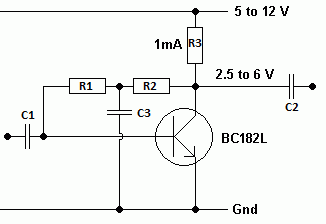RANDOM PAGE

SITE SEARCH

LOG
IN

HELP

# Common Emitter Amplifier

This is the AQA version closing after June 2019. Visit the the version for Eduqas instead.This can be used as an audio pre-amplifier. Normally an op amp would be used. This does not need a negative supply and could work on lower voltages than an op amp needs. A field effect transistor would have a higher input resistance.

For a 12 volt supply ...

• The collector voltage should be 6 volts. Calculate R3.
• For 1 mA to flow ...
• R3 = V / I = 6 / 0.001 = 6kΩ.
• A typical BC182L has a current gain of 300 so the base current needs to be 1/300 mA = 3.3 µA.
• Calculate R1 + R2.
• The voltage across this pair is 6 - 0.7 = 5.3 Volts.
• R = V / I = 5.3 / 3.3x10-6 = 1.6MΩ.
• R1 = R2 = 800 kΩ.
• C1 = 470 nF
• C2 = 10 µF
• C3 = 20 nF

reviseOmatic V3     Contacts, ©, Cookies, Data Protection and Disclaimers Hosted at linode.com, London